It’s World Statistics Day! To honor the theme of the day, the JMP User Community is having conversations about the importance of trust in statistics and data. And we want to hear from you! Tell us the steps you take to ensure that your data is trustworthy.
Choose Language Hide Translation Bar
Highlighted

## How to create a CDF plot without using the Fit Y by X platform interactively?

hi, Here is an example, using big class data table. In order to get the CDF plot, I have to do a y by x and enable CDF option to get it. My question is how to plot CDF plot without doing y by x plot? I scratched my hair very hard, no vial.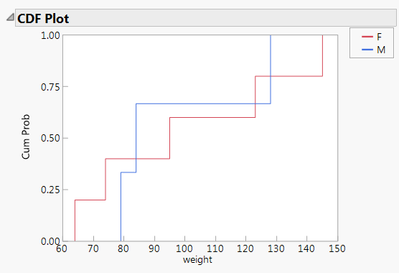Oneway(

SendToByGroup( Bygroup Default ),

Y( :weight ),

SendToByGroup( {:age == 12}, Y( :weight ) ),

X( :sex ),

SendToByGroup( {:age == 12}, X( :sex ), CDF Plot( 1 ) ),

By( :age ),

SendToByGroup(

{:age == 12},

SendToReport(

Dispatch(

{"Oneway Analysis of weight By sex age=12"},

"CDF Plot",

OutlineBox,

{SetHorizontal( 1 )}

)

)

)

);

1 ACCEPTED SOLUTION

Accepted Solutions
Highlighted

## Re: how to plot CDF plot without doing y by x plot

I am a little confused about your statement that it is hard to make a script that uses the Distribution or Oneway platform to create a normal quantile plot or a CDF plot.  Are you looking for a separate function that generates such a plot? You can generate a Graph Box, and then place into it any graphics you want.  But you would have to generate the Normal quantile or CDF plots from scratch.

I am also wondering if you are not aware that all you have to do to create such a script, is to interactively create the display output using the platform you want, and then to go to the red triangle==>script==>Write Script to Script Window.  It generates the exact code you need to generate the normal quantile of CDF plot, or whatever else you have specified.

Is this getting closer to what you need?

Jim
6 REPLIES 6
Highlighted

## Re: how to plot CDF plot without doing y by x plot

Under distribution there is a CDF plot.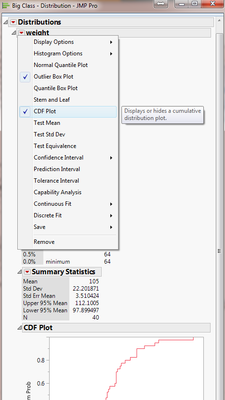Vince Faller - Predictum
Highlighted

## Re: how to plot CDF plot without doing y by x plot

Let me rephrase my question, I would like be able to script a normal quantile plot or a CDF plot. I knew we can do it by doing distribution or oneway analysis but it is make very hard to script.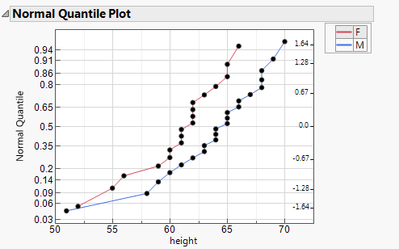Highlighted

## Re: how to plot CDF plot without doing y by x plot

I am a little confused about your statement that it is hard to make a script that uses the Distribution or Oneway platform to create a normal quantile plot or a CDF plot.  Are you looking for a separate function that generates such a plot? You can generate a Graph Box, and then place into it any graphics you want.  But you would have to generate the Normal quantile or CDF plots from scratch.

I am also wondering if you are not aware that all you have to do to create such a script, is to interactively create the display output using the platform you want, and then to go to the red triangle==>script==>Write Script to Script Window.  It generates the exact code you need to generate the normal quantile of CDF plot, or whatever else you have specified.

Is this getting closer to what you need?

Jim
Highlighted

## Re: how to plot CDF plot without doing y by x plot

hi, Jim,

Yes, I am looking for a separate function that generates such a plot so that I could iterate it in a loop to plot the plots one by one and output it into a journal, then save a .png. The situation that normal qualtiles  plot is embedded in either oneway or distribution make it difficult for me to enable it (my skill in scripting).

With your helps, I am able to make it just plot the normal quantile. I do not know how to change the title of the plot though.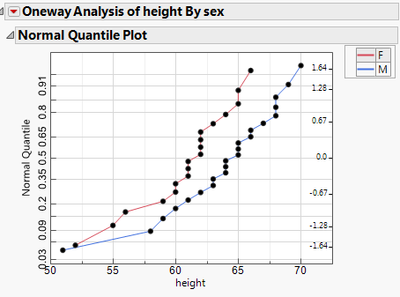Oneway(

Y( :height ),

X( :sex ),

All Graphs( 0 ),

Plot Quantile by Actual( 1 ),

Line of Fit( 0 ),

Mean Diamonds( 0 ),

X Axis Proportional( 0 ),

Grand Mean( 0 ),

SendToReport(

Dispatch(

{"Normal Quantile Plot"},

"2",

ScaleBox,

{Label Row( Label Orientation( "Vertical" ) )}

)

)

);

Highlighted

## Re: how to plot CDF plot without doing y by x plot

I will first deal with the figuring out, of how to change the Title of the Plot.  I assume, what you are referring to, is the Title of the Outline Box that has the Title of "Normal Quantile Plot".  The simple answer to this is that you change it interactively, and then have JMP generate the script.  In the script, you will see the method to change the title.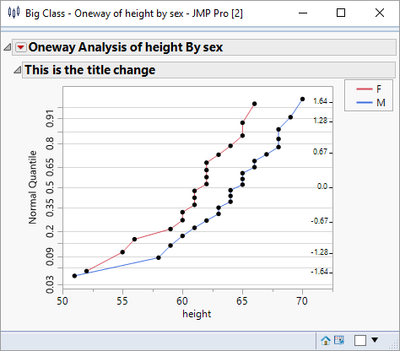Here is the script that JMP generated that will produce the change in the title

Oneway(

Y( :height ),

X( :sex ),

All Graphs( 0 ),

Plot Quantile by Actual( 1 ),

Line of Fit( 0 ),

X Axis Proportional( 0 ),

Grand Mean( 0 ),

SendToReport(

Dispatch(

{},

"Normal Quantile Plot",

OutlineBox,

{Set Title( "This is the title change" )}

),

Dispatch(

{"Normal Quantile Plot"},

"2",

ScaleBox,

{Label Row( Label Orientation( "Vertical" ) )}

)

)

);

So given that, you can turn the title into a JMP variable, and just use it in the above structure

MyTitle = "Here is a new Change";

Oneway(

Y( :height ),

X( :sex ),

All Graphs( 0 ),

Plot Quantile by Actual( 1 ),

Line of Fit( 0 ),

X Axis Proportional( 0 ),

Grand Mean( 0 ),

SendToReport(

Dispatch(

{},

"Normal Quantile Plot",

OutlineBox,

{Set Title( MyTitle)}

),

Dispatch(

{"Normal Quantile Plot"},

"2",

ScaleBox,

{Label Row( Label Orientation( "Vertical" ) )}

)

)

);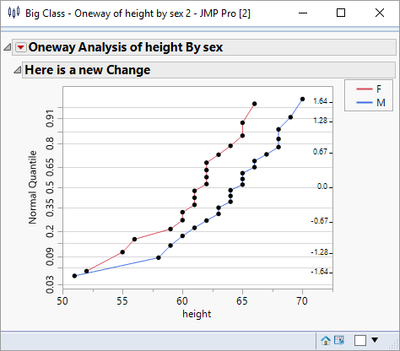Now, lets deal with the generation of all of the graphs.  Using the Big Class data table, there are really only 2 columns to be graphed, but that is enough to illustrate the method.

Names Default To Here( 1 );

dt = Open( "\$SAMPLE_DATA/Big Class.jmp" );

// Get the continuous columns from the data table

ColList = dt << get column names( continuous );

// Loop across the list of columns and produce a graph for each column

For( i = 1, i <= N Items( ColList ), i++,

MyTitle = "Here is a new Change " || char(i);

Oneway(

Y( ColList[i] ),

X( :sex ),

All Graphs( 0 ),

Plot Quantile by Actual( 1 ),

Line of Fit( 0 ),

X Axis Proportional( 0 ),

Grand Mean( 0 ),

SendToReport(

Dispatch( {}, "Normal Quantile Plot", OutlineBox, {Set Title( MyTitle )} ),

Dispatch( {"Normal Quantile Plot"}, "2", ScaleBox, {Label Row( Label Orientation( "Vertical" ) )} )

)

);

);

It produces a separate display for each Normal Quantile Plot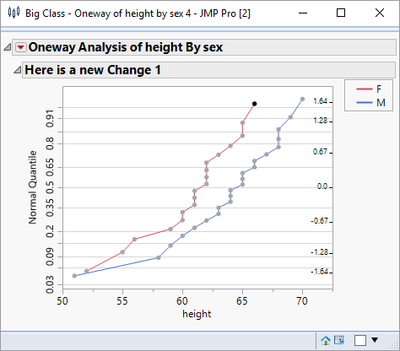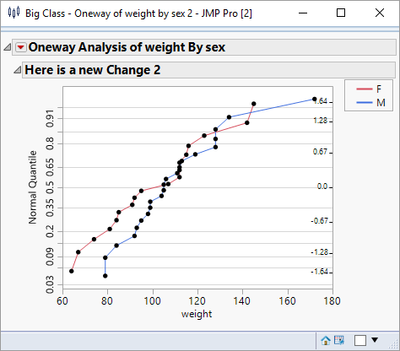So the next step will put them all together in one window

Names Default To Here( 1 );

dt = Open( "\$SAMPLE_DATA/Big Class.jmp" );

// Get the continuous columns from the data table

ColList = dt << get column names( continuous );

// Create your own display window and generate the graph inside

New Window( "Here are the graphs",

// Now I am choosing to put all of the graphs side by side so I am

// using an H List Box() to encaptulate the graphs

H List Box(

// Loop acrss the list of columns and produce a graph for each column

For( i = 1, i <= N Items( ColList ), i++,

MyTitle = "Here is a new Change " || Char( i );

Oneway(

Y( ColList[i] ),

X( :sex ),

All Graphs( 0 ),

Plot Quantile by Actual( 1 ),

Line of Fit( 0 ),

X Axis Proportional( 0 ),

Grand Mean( 0 ),

SendToReport(

Dispatch( {}, "Normal Quantile Plot", OutlineBox, {Set Title( MyTitle )} ),

Dispatch( {"Normal Quantile Plot"}, "2", ScaleBox, {Label Row( Label Orientation( "Vertical" ) )} )

)

);,

)

)

);

Which produces: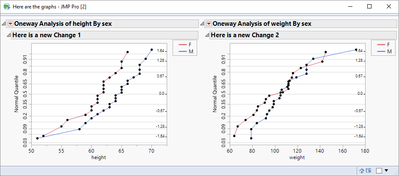Jim
Highlighted

## Re: how to plot CDF plot without doing y by x plot

hi, Jim

Where I wanted to change is :"Oneway Analysis".